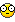# need help with cos,sin and tan

## Recommended Posts

how do i use them with autoit.

what we have in school was to calculate the degree of one of the corners when one of degrees are 90* and we know the lenght of the side's

the problem is that i cant get the right number's of degree's in the corner i tryed to find.

```#include <GUIConstants.au3>

\$pi = 3.14159265358979

\$Form1 = GUICreate("", 390, 300, 193, 115)
\$Input5 = GUICtrlCreateInput("hyp",150,80,50)
\$Input6 = GUICtrlCreateInput("1",315,130,50)
\$Input7 = GUICtrlCreateInput("2",150,210,50)
\$Input9 = GUICtrlCreateInput("Degree",100,170,50)
\$Button3 =  GUICtrlCreateButton("calculate", 280, 270, 80, 20)
\$box1gui = GUICreate("", 320,280,20,-20,\$WS_POPUP,BitOr(\$WS_EX_LAYERED,\$WS_EX_MDICHILD),\$Form1)
GUICtrlCreatePic("triangle.gif",0,0,320,280)

GUISetState(@SW_SHOW,\$Form1)
GUISetState(@SW_SHOW,\$box1gui)
Do
\$nmsg = GUIGetMsg()
if \$nmsg = \$Button3 then calculate()
until \$nmsg = \$GUI_EVENT_CLOSE

func calculate()
if \$1 = "" Then
EndIf
EndFunc```

really need some help with this, normaly when i use my calculator i just hit cos(\$1/\$hyp) or cos-1(\$1/\$hyp) cant rememberPS:

whould also like to know how i could calculate the lenght of one of the side's when i know the degree's and one of the other side's

i hate this kind of math

sry for if you cant understand what im asking for.

[font="Impact"]Never fear, I is here.[/font]

##### Share on other sites

Only syntax correction, no math logic added:

```func calculate()
;~     if \$1 = "" Then
;~     EndIf
EndFunc```

So now it's generating some numbers ...

##### Share on other sites

This is the basic idea, but careful because I haven't checked it which means there are probably mistakes.

```#include <GUIConstants.au3>

\$pi = 3.14159265358979

\$Form1 = GUICreate("", 390, 300, 193, 115)
\$Input5 = GUICtrlCreateInput("hyp",150,80,50)
\$Input6 = GUICtrlCreateInput("1",315,130,50)
\$Input7 = GUICtrlCreateInput("2",150,210,50)
\$Input9 = GUICtrlCreateInput("Degree",100,170,50)
\$Button3 =  GUICtrlCreateButton("calculate", 280, 270, 80, 20)
\$box1gui = GUICreate("", 320,280,20,-20,\$WS_POPUP,BitOr(\$WS_EX_LAYERED,\$WS_EX_MDICHILD),\$Form1)
GUICtrlCreatePic("triangle.gif",0,0,320,280)

GUISetState(@SW_SHOW,\$Form1)
GUISetState(@SW_SHOW,\$box1gui)
Do
\$nmsg = GUIGetMsg()
if \$nmsg = \$Button3 then calculate()
until \$nmsg = \$GUI_EVENT_CLOSE

func calculate()

if \$a = '' Then
if \$1 <> '' and \$2 <> '' Then

Elseif \$1 <> '' and \$hyp <> '' Then

elseif \$2 <>'' and \$hyp <> '' Then

EndIf

Else
if \$1 = '' and \$hyp <> '' Then

Elseif \$2 = "" and \$hyp <> '' Then

Elseif \$hyp = '' and \$1 <> ''  Then

ElseIf \$hyp = '' and \$2 <> '' Then
EndIf
EndIf

EndFunc```
Serial port communications UDF Includes functions for binary transmission and reception.printing UDF Useful for graphs, forms, labels, reports etc.Add User Call Tips to SciTE for functions in UDFs not included with AutoIt and for your own scripts.Functions with parameters in OnEvent mode and for Hot Keys One function replaces GuiSetOnEvent, GuiCtrlSetOnEvent and HotKeySet.UDF IsConnected2 for notification of status of connected state of many urls or IPs, without slowing the script.
##### Share on other sites

PS:

whould also like to know how i could calculate the lenght of one of the side's when i know the degree's and one of the other side's

i hate this kind of math

sry for if you cant understand what im asking for.

sin theta = opposite / hypotenuse

cos theta = adjacent / hypotenuse

tan theta = opposite / adjacent

Say you have a 30 degree angle and opposite side length is 3 and you want to know the length of the adjacent side (bottom).

Use t for tangent of 30 degrees since tan = opp/adj a relationship of the sides in which we are interested.

t = 3/x

xt = 3

x = 3/t

since tan of 30 degrees = .577

x = 3 / 0.577

x = 5.19

~~~~~~~~~~~~~~

\$pi = 3.14159265

\$angle = 30

\$opp = 3

\$adjacent = \$opp/(tan (\$angle * \$d2R))

## Create an account

Register a new account

• ### Recently Browsing   0 members

×

• Wiki

• Back

• #### Beta

• Git
• FAQ
×
• Create New...